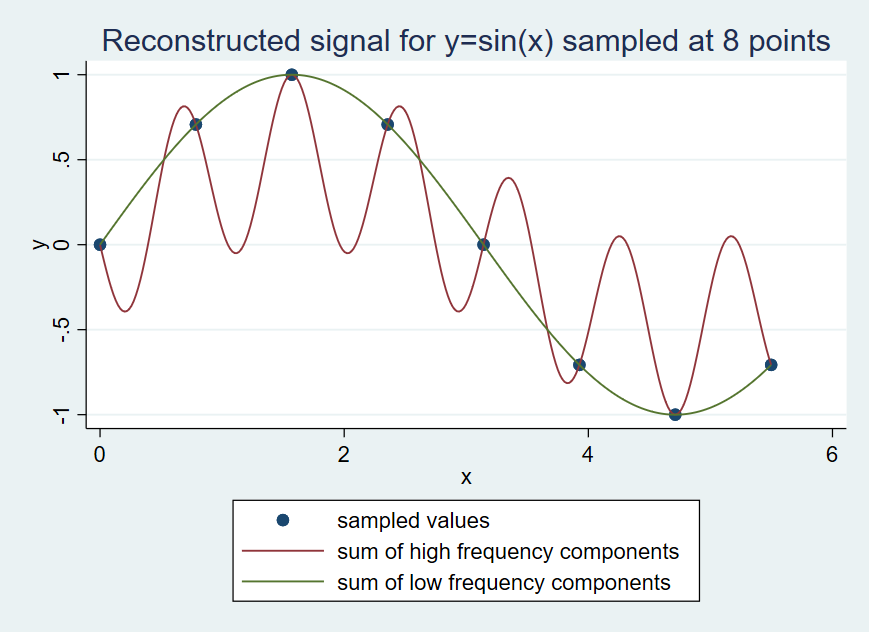Search

# Graph Fourier Transform in Stata

You can create a graph for fourier transform in Stata. An example is shown below:To create the graph above, please use the following commands in a do file:

```clear
set obs 8
gen x=(_n-1)*(2*_pi)/8
gen y=sin(x)
mata: h=st_data(.,"y")
mata: fft(h)
# delimit ;
twoway scatter y x || function y=1/8*(4*sin(1*x)-4*sin( 7*x)), range(x) ///
|| function y=1/8*(4*sin(1*x)-4*sin(-1*x)), range(x) ///
||, legend(cols(1) label(1 "sampled values") ///
label(2 "sum of high frequency components") ///
label(3 "sum of low frequency components")) ///
title("Reconstructed signal for y=sin(x) sampled at 8 points");
# delimit cr```

You may find more useful tips in the book Speaking Stata Graphics